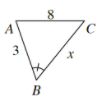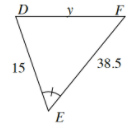### Home > CCAA > Chapter 9 Unit 10 > Lesson CC3: 9.1.4 > Problem9-45

9-45.

$ΔABC$ is similar to $ΔDEF$.

1. Find the scale factor from $ΔABC$ to $ΔDEF$.

Find the scale factor between two given similar sides for the two shapes.

The scale factor is $5$.

2. Find $x$.

Divide the side corresponding to side $x$ by the scale factor.

$x=\frac{38.5}{5}$

3. Find $y$.

Multiply the matching side by the scale factor.